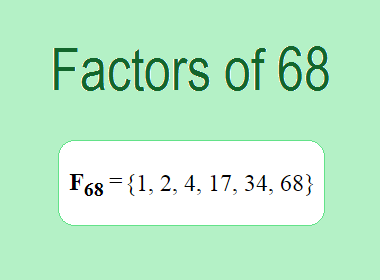# Factors of 68The factors of 68 are 1, 2, 4, 17, 34, and 68 i.e. F68 = {1, 2, 4, 17, 34, 68}. The factors of 68 are all the numbers that can divide 68 without leaving a remainder.

We can check if these numbers are factors of 68 by dividing 68 by each of them. If the result is a whole number, then the number is a factor of 68. Let's do this for each of the numbers listed above:

·        1 is a factor of 68 because 68 divided by 1 is 68.

·        2 is a factor of 68 because 68 divided by 2 is 34.

·        4 is a factor of 68 because 68 divided by 4 is 17.

·        17 is a factor of 68 because 68 divided by 17 is 4.

·        34 is a factor of 68 because 68 divided by 34 is 2.

·        68 is a factor of 68 because 68 divided by 68 is 1.

## How to Find Factors of 68?

1 and the number itself are the factors of every number. So, 1 and 68 are two factors of 68. To find the other factors of 68, we can start by dividing 68 by the numbers between 1 and 68. If we divide 68 by 2, we get a remainder of 0. Therefore, 2 is a factor of 68. If we divide 68 by 3, we get a remainder of 2. Therefore, 3 is not a factor of 68.

Next, we can check if 4 is a factor of 68. If we divide 68 by 4, we get a remainder of 0. Therefore, 4 is also a factor of 68. We can continue this process for all the possible factors of 68.

Through this process, we can find that the factors of 68 are 1, 2, 4, 17, 34, and 68. These are the only numbers that can divide 68 without leaving a remainder.

********************

********************

## Properties of the Factors of 68

The factors of 68 have some interesting properties. One of the properties is that the sum of the factors of 68 is equal to 126. We can see this by adding all the factors of 68 together:

1 + 2 + 4 + 17 + 34 + 68 = 126

Another property of the factors of 68 is that the prime factors of 68 are 2, and 17 only.

## Applications of the Factors of 68

The factors of 68 have several applications in mathematics. One of the applications is in finding the highest common factor (HCF) of two or more numbers. The HCF is the largest factor that two or more numbers have in common. For example, to find the HCF of 68 and 51, we need to find the factors of both numbers and identify the largest factor they have in common. The factors of 68 are 1, 2, 4, 17, 34, and 68. The factors of 51 are 1, 3, 17, and 51. The largest factor that they have in common is 17. Therefore, the HCF of 68 and 51 is 17.

Another application of the factors of 68 is in prime factorization. Prime factorization is the process of expressing a number as the product of its prime factors. The prime factors of 68 are 2, and 17 since these are the only prime numbers that can divide 68 without leaving a remainder. Therefore, we can express 68 as:

68 = 2 × 2 × 17

We can do prime factorization by division and factor tree method also. Here is the prime factorization of 68 by division method,

Image 1

68 = 2 × 2 × 17

Here is the prime factorization of 68 by the factor tree method,

Image 2

68 = 2 × 2 × 17

## Conclusion

The factors of 68 are the numbers that can divide 68 without leaving a remainder. The factors of 68 are 1, 2, 4, 17, 34, and 68. The factors of 68 have some interesting properties, such as having a sum of 126. The factors of 68 have several applications in mathematics, such as finding the highest common factor and prime factorization.